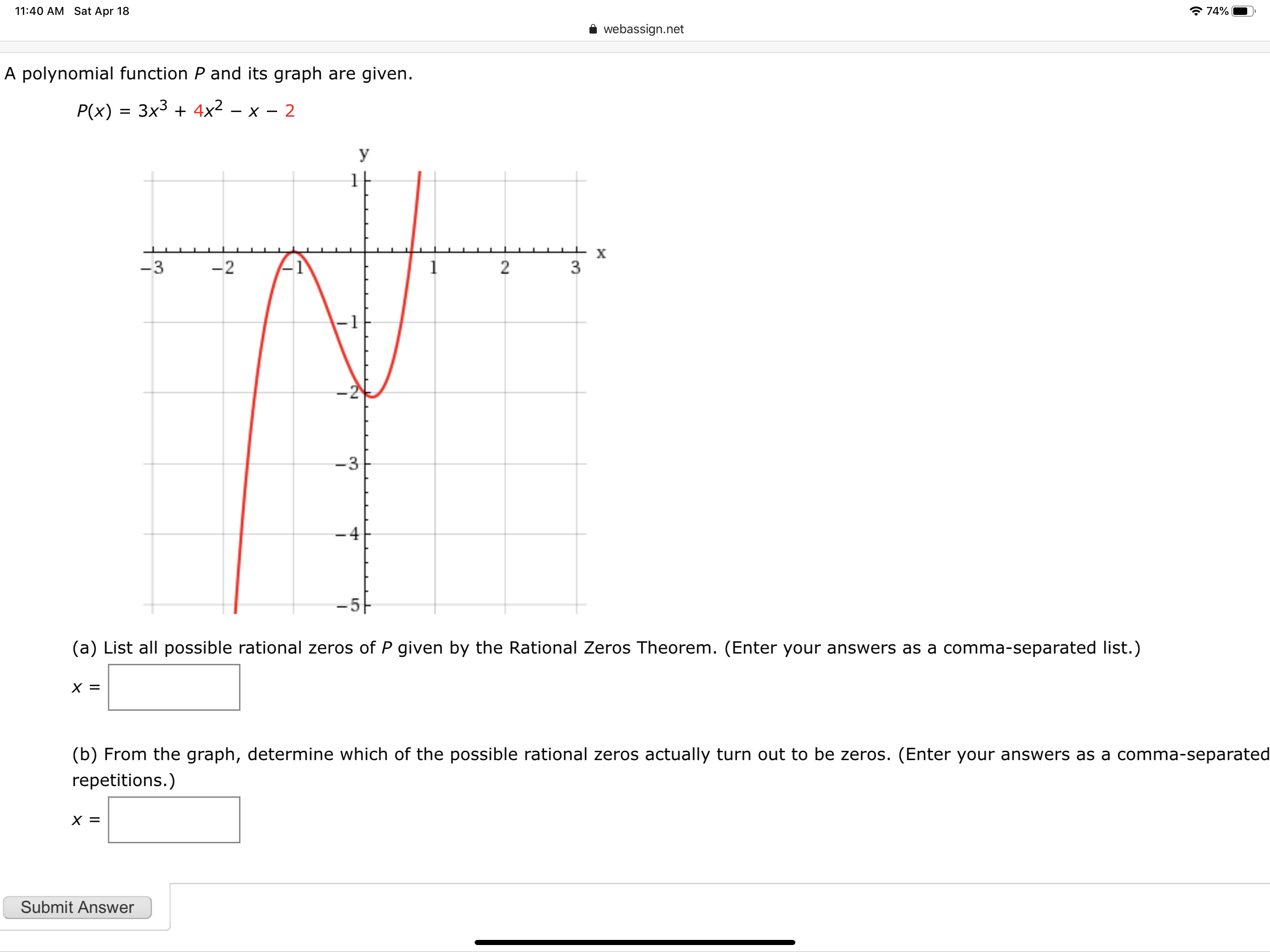# 11:40 AM Sat Apr 18 * 74% webassign.net A polynomial function P and its graph are given. P(x) = 3x3 + 4x2 – x – 2 У х -3 -2 -4 (a) List all possible rational zeros of P given by the Rational Zeros Theorem. (Enter your answers as a comma-separated list.) (b) From the graph, determine which of the possible rational zeros actually turn out to be zeros. (Enter your answers as a comma-separated repetitions.) Submit Answer 3. 2. 3.

Questionhelp_outlineImage Transcriptionclose11:40 AM Sat Apr 18 * 74% webassign.net A polynomial function P and its graph are given. P(x) = 3x3 + 4x2 – x – 2 У х -3 -2 -4 (a) List all possible rational zeros of P given by the Rational Zeros Theorem. (Enter your answers as a comma-separated list.) (b) From the graph, determine which of the possible rational zeros actually turn out to be zeros. (Enter your answers as a comma-separated repetitions.) Submit Answer 3. 2. 3. fullscreen

### Want to see this answer and more?

Experts are waiting 24/7 to provide step-by-step solutions in as fast as 30 minutes!*

*Response times may vary by subject and question complexity. Median response time is 34 minutes for paid subscribers and may be longer for promotional offers.
Tagged in
Math
Calculus

### Functions